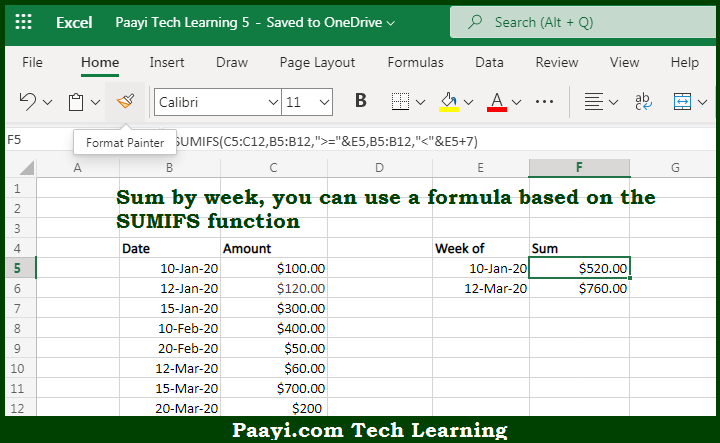# Learn How to SUM By Week in Microsoft Excel

Written by | 0 Comments | 565 Views

In this article, you will learn how to COUNT various things in Microsoft Excel using a single/combination(s) of functions. You will also know how to SUM By Week and see the generic formula.

SUM By Week in Microsoft Excel

The main purpose of this formula is to sum by week. Here we will learn how to sum by provided week in the workbook in Microsoft Excel. That implies, with the help of a formula based on the SUMIFS function you can able sum by week. So, with the help of this formula, you can able to sum by provided week in the workbook in Microsoft Excel.

General Formula to SUM By Week

=SUMIFS(value,date,">="&A1,date,"<"&A1+7)

The Explanation for the SUM By WeekSo we know that with the help of the given formula above you can able to sum by week. Here we will learn how to sum by provided week in the workbook in Microsoft Excel. As we know that the SUMIFS function can sum ranges based on many numbers of criteria. In the example given above, the SUMIFS function is used to sum amounts by week using two criteria - first the dates greater than or equal to the date in column E, and secondly dates less than the date in column E plus 7 days. And when this formula is used, the SUMIFS functions generate a sum for each week So, with the help of this formula, you can able to sum by provided week in the workbook in Microsoft Excel.